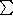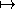## CGAL::compose

### Definition

The function compose is used to compose functors f0,...,fn into each other, thereby creating a new functor f. The first argument f0 always denotes the base functor, for which the remaining functors f1,...,fn provide the arguments. If we denote the arity of a functor f by ar(f), then ar(f) =i=1n ar(fi), i.e., the arguments of f are distributed among f1,...,fn according to their respective arity. Between one and three functors can be composed into the base functor, giving raise to a functor of arity at most five.

As an example, consider a binary functor f0 and two functors f1 and f2, with arity three and two, respectively. Composing f1 and f2 into f0 yields a new functor

 f:(x0,x1,x2,x3,x4)f0(f1(x0,x1,x2),f2(x3,x4))

with arity five.

#include <CGAL/functional.h>

 template < class F0, class F1 > typename Compose< F0, F1 >::Type compose ( F0 f0, F1 f1) returns the functor f0(f1( · )) with the same arity as f1. Requirement: f0 is unary function (arity 1). f0 and f1 are models for AdaptableFunctor. template < class F0, class F1, class F2 > typename Compose< F0, F1, F2 >::Type compose ( F0 f0, F1 f1, F2 f2) returns the functor f0(f1( · ),f2( · )) with arity equal to ar(f1)+ar(f2). Requirement: f0 is binary function (arity 2). f0, f1, and f2 are models for AdaptableFunctor. template < class F0, class F1, class F2, class F3 > typename Compose< F0, F1, F2, F3 >::Type compose ( F0 f0, F1 f1, F2 f2, F3 f3) returns the functor f0(f1( · ),f2( · ),f3( · )) with arity equal to ar(f1)+ar(f2)+ar(f3). Requirement: f0 is ternary function (arity 3). f0, f1, f2, and f3 are models for AdaptableFunctor.